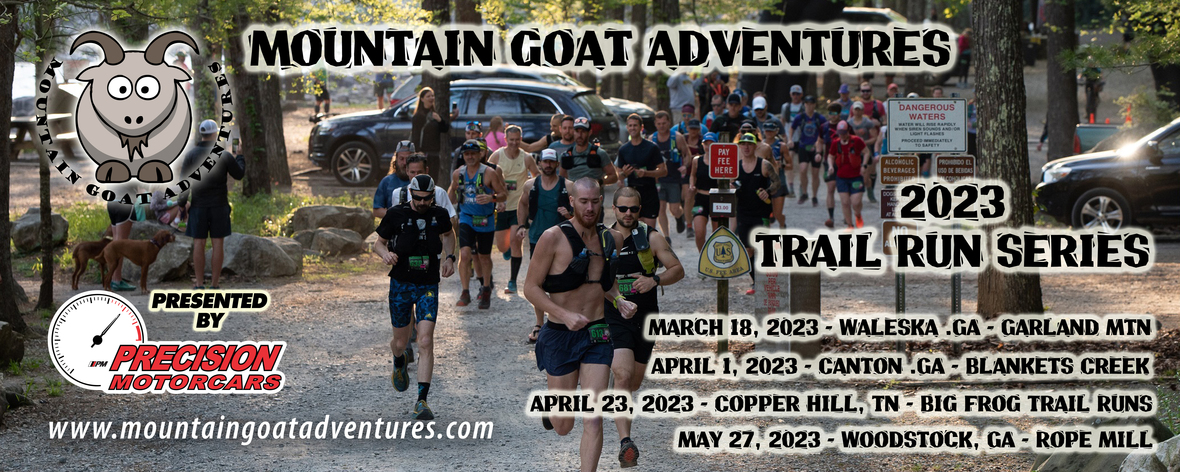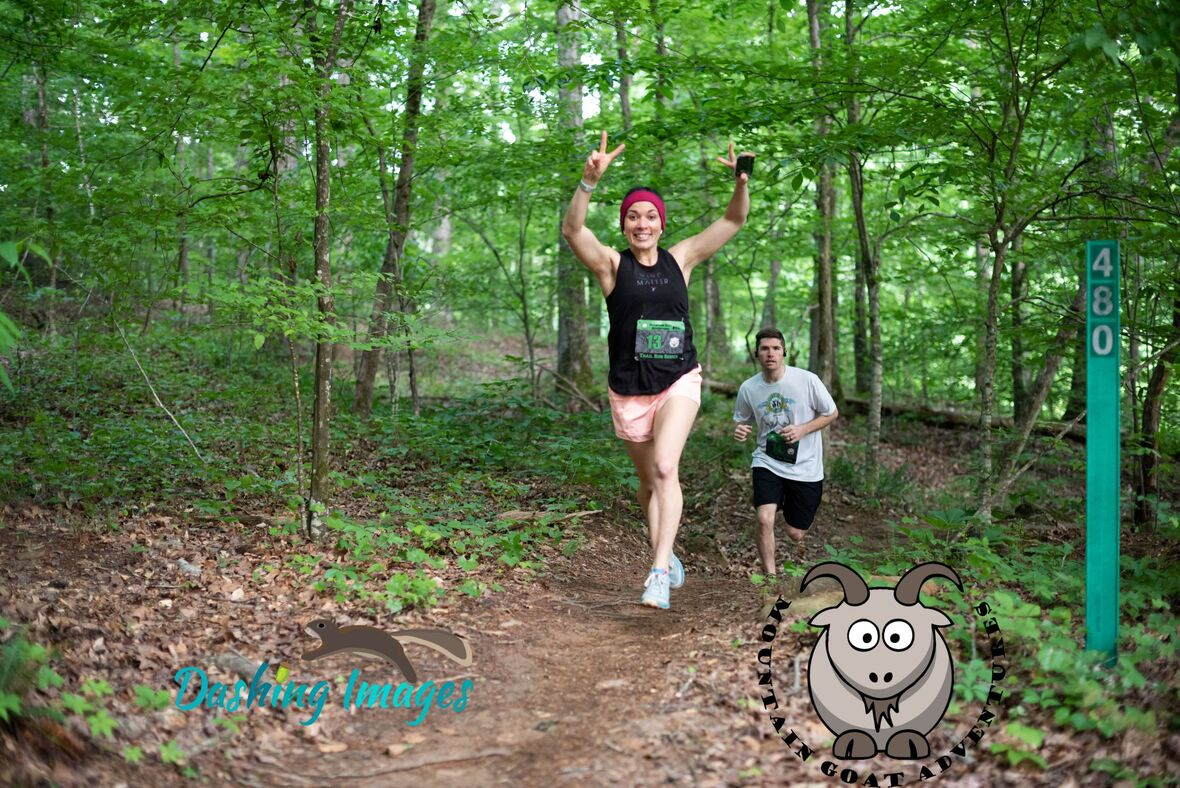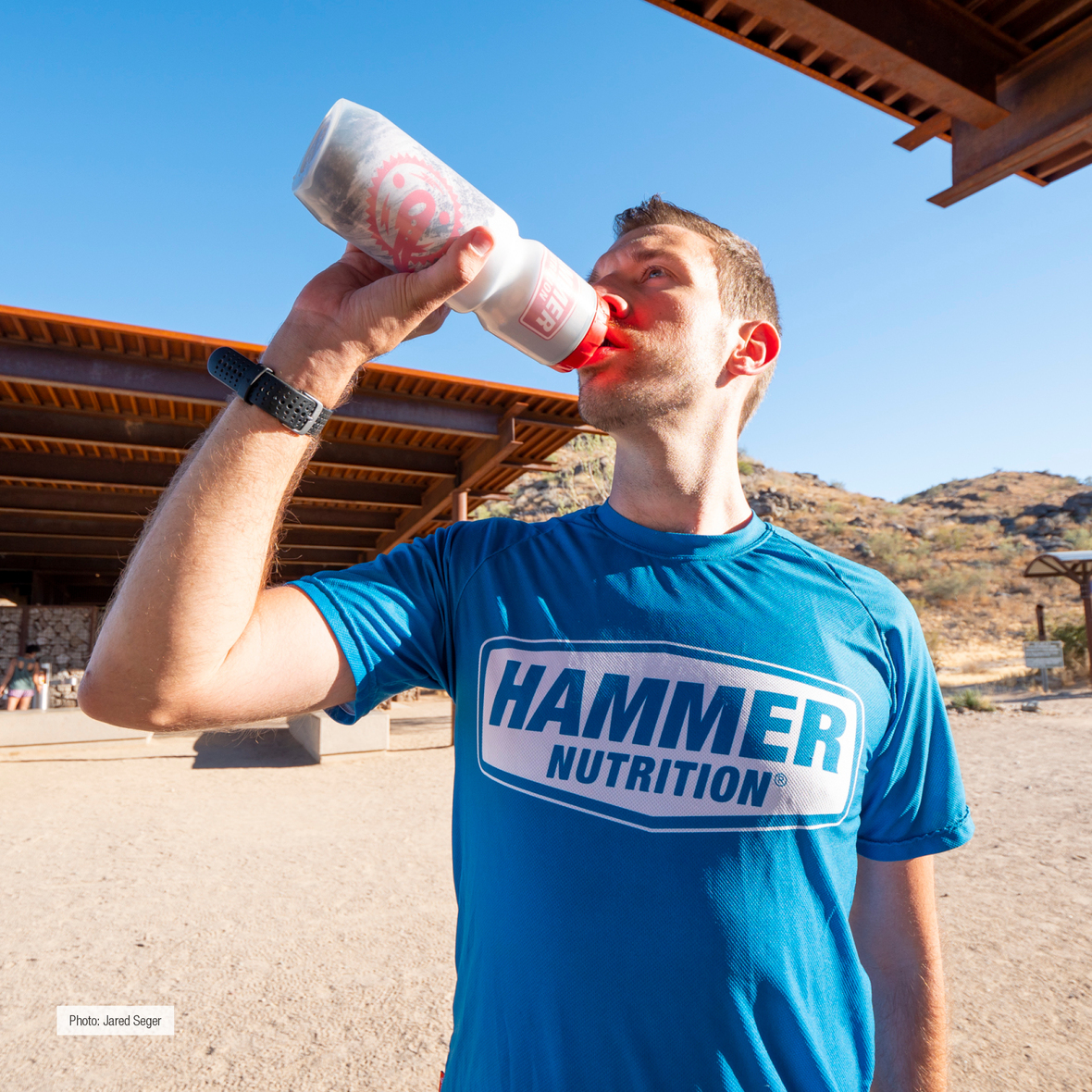# KEY DETAILS YOU NEED TO KNOW/ACTION ITEMS:

▪ OPTIONAL early check-in Thursday May 25th from 4 to 7 pm at the new Fleet Feet Canton, 1431 Riverstone Pkwy, Canton, GA 30114. If you check in early, you can go straight to the start line on Saturday morning. Fleet Feet is also offering 10% off items purchased during packet pickup.
▪ Waivers need to be printed at home, filled out ahead of time, and brought to the race check-in CLICK HERE FOR EVENT WAIVER
▪ No race day distance changes - if you want to change distance, please contact me via email now and I'll get you taken care of. No changes after 5/22.
▪ If you can no longer attend, you may sell your entry to someone else, but this needs to be done by 5/22. Payment is between buyer and seller..all I need is the new runner's name and email no later than 5/22. No late transfers, and no transfers to other events at this point.
▪ SCHEDULE: 8 am - Half Marathon Start, 8:05 - 10K Start
 ▪ OPTIONAL early check-in Thursday May 25th from 4 to 7 pm at the new Fleet Feet Canton, 1431 Riverstone Pkwy, Canton, GA 30114. If you check in early, you can go straight to the start line on Saturday morning. Fleet Feet is also offering 10% off items purchased during packet pickup.
 ▪ Waivers need to be printed at home, filled out ahead of time, and brought to the race check-in CLICK HERE FOR EVENT WAIVER
 ▪ No race day distance changes - if you want to change distance, please contact me via email now and I'll get you taken care of. No changes after 5/22.
 ▪ If you can no longer attend, you may sell your entry to someone else, but this needs to be done by 5/22. Payment is between buyer and seller..all I need is the new runner's name and email no later than 5/22. No late transfers, and no transfers to other events at this point.
 ▪ SCHEDULE: 8 am - Half Marathon Start, 8:05 - 10K Start

# We have plenty of room left in this event if you have friends who'd like to join us! Registration is open until 5pm on May 26th!

## CHECK-IN

▪ Saturday Check-in hours are from 6:30 am to 7:45 am. When you arrive, parking helpers will direct you where to park - please park where directed. Overflow parking is at Kirk Rudy, which is a half mile away. Plan ahead and bring a backpack if you have to park there. You can leave any bags in the pavilion while you race.
▪ Proceed to appropriate check-in line with waiver. Hand sanitizer will be available.
▪ Port-o-lets as well as permanent bathrooms with running water will be available at this venue.
 ▪ Saturday Check-in hours are from 6:30 am to 7:45 am. When you arrive, parking helpers will direct you where to park - please park where directed. Overflow parking is at Kirk Rudy, which is a half mile away. Plan ahead and bring a backpack if you have to park there. You can leave any bags in the pavilion while you race.
 ▪ Proceed to appropriate check-in line with waiver. Hand sanitizer will be available.
 ▪ Port-o-lets as well as permanent bathrooms with running water will be available at this venue.

## RACE PROCEDURES

▪ Make sure your number is pinned on your front - do not bend the foam timing chip. Number belts are fine, just make sure it is on your front as you pass over the timing mats. Your time starts when the horn goes off (gun time).
▪ Brief pre-race meeting at 7:45 am near the pavilion.
▪ 10K runners will pass the an aid station at Mile 3.4 along the Avalache Trail.
▪ Half Marathon runners will have an aid station available at Mile 5, 8.3 and 11.1. Aid station will have water, HEED, and Hammer gels. If you take anything from the aid station, you MUST place it in the trash - cups need to be fully emptied before being put in the trash. Please, no throwing cups or trash on the ground. Hand sanitizer will be available at the aid station. Do not throw trash anywhere on the course - penalty is DQ.
▪ Course will be well marked with pink ribbons and pink/black arrow signs at turns. Pink ribbons indicate the direction you want to head and are also used to designate that you are on-course if it has been a while in between turns. Course marshals will be positioned throughout the course for your safety.
▪ ANNOUNCE if you are passing to runners ahead and say PASSING RIGHT or PASSING LEFT and give them a chance to move to the side. PLEASE BE PATIENT, WORK TOGETHER, BE NICE and give each other space out on the trails.
 ▪ Make sure your number is pinned on your front - do not bend the foam timing chip. Number belts are fine, just make sure it is on your front as you pass over the timing mats. Your time starts when the horn goes off (gun time).
 ▪ Brief pre-race meeting at 7:45 am near the pavilion.
 ▪ 10K runners will pass the an aid station at Mile 3.4 along the Avalache Trail.
 ▪ Half Marathon runners will have an aid station available at Mile 5, 8.3 and 11.1. Aid station will have water, HEED, and Hammer gels. If you take anything from the aid station, you MUST place it in the trash - cups need to be fully emptied before being put in the trash. Please, no throwing cups or trash on the ground. Hand sanitizer will be available at the aid station. Do not throw trash anywhere on the course - penalty is DQ.
 ▪ Course will be well marked with pink ribbons and pink/black arrow signs at turns. Pink ribbons indicate the direction you want to head and are also used to designate that you are on-course if it has been a while in between turns. Course marshals will be positioned throughout the course for your safety.
 ▪ ANNOUNCE if you are passing to runners ahead and say PASSING RIGHT or PASSING LEFT and give them a chance to move to the side. PLEASE BE PATIENT, WORK TOGETHER, BE NICE and give each other space out on the trails.

## RESULTS/AWARDS

▪ Finish times wlll be available as you finish on tablets at our results tent. Check your result, make sure you're in the correct category, etc. Medals awarded to the top 3 in each age group. *10K awards at approximately 9:30 am, short course series awards to follow, and half marathon awards at 10:30 am, long course series awards to follow, near the pavilions. AWARDS WILL NOT BE MAILED - THEY MUST BE PICKED UP AT THE RACE
 ▪ Finish times wlll be available as you finish on tablets at our results tent. Check your result, make sure you're in the correct category, etc. Medals awarded to the top 3 in each age group. *10K awards at approximately 9:30 am, short course series awards to follow, and half marathon awards at 10:30 am, long course series awards to follow, near the pavilions. AWARDS WILL NOT BE MAILED - THEY MUST BE PICKED UP AT THE RACEtable div table+table+table+table+table+table+table div table{width:100%;padding:0}table div table+table+table+table+table+table+table div table img{width:96.23%;padding:0;float:none}table div table+table+table+table+table+table+table div table td{width:100%;padding:0 1.88% 18px}/* styles */## Early packet pickup hosted by Fleet-Feet Canton!

 table div table+table+table+table+table+table+table+table+table div table,table.module-8{width:52.83%;float:left;padding:0}table div table+table+table+table+table+table+table+table+table div table a{border:0 none;text-decoration:none}table div table+table+table+table+table+table+table+table+table div table img{width:100%!important;border:0 none;text-decoration:none}table div table+table+table+table+table+table+table+table+table div table td{width:100%;padding:0 20px 20px 0}/* styles */ We are excited to be partnering with Canton's newest running store - Fleet Feet Canton. They are located at 1431 Riverstone Pkwy, Canton, GA 30114. They will be hosting our early packet pickup on Thursday May 25th from 4-7 pm. 10% off all purchases during packet pickup and 10% off with your race bib after the race! Fleet Feet carries some of my favorite brands including Altra, Vuori, and Lululemon!
 table div table+table+table+table+table+table+table+table+table+table div table{width:100%;padding:0}table div table+table+table+table+table+table+table+table+table+table div table img{width:96.23%;padding:0;float:none}table div table+table+table+table+table+table+table+table+table+table div table td{width:100%;padding:0 1.88% 18px}/* styles */## Fueling Tips from Hammer NutritionYour body needs to run at peak capacity so you can get your best result during the race. Here are a few tips from Hammer Nutrition to get started and make sure your body runs at peak capacity from start to finish!

Tip #1 Hydration: Replenish, don’t replace! Keep fluid intake below 25 oz./hr during exercise. FIND OUT HOW MUCH

Tip #2 Caloric Intake: Stay fueled, but not overwhelmed, by consuming 120-180 calories/hr. ARE CARBS BEST?

Tip #3 Electrolytes: You need an array of vitamins and minerals, not just salt, to finish strong. WHAT DO YOU NEED?

Tip #4 Pre-Event Fueling: Don’t tax your system with a meal within 3 hours of start time. BUT WHAT IF YOU'RE HUNGRY?

Tip #5 Recovery: Refuel your tank with 60 minutes of finishing a workout. ARE CARBS OR PROTEIN BETTER?

 table div table+table+table+table+table+table+table+table+table+table+table+table+table div table{width:100%;padding:0}table div table+table+table+table+table+table+table+table+table+table+table+table+table div table img{width:96.23%;padding:0;float:none}table div table+table+table+table+table+table+table+table+table+table+table+table+table div table td{width:100%;padding:0 1.88% 18px}/* styles */## Volunteer Opportunities and Trail Work Parties

We are partnering with SORBA Woodstock to bring you these events. SORBA Woodstock has built and maintains the trails at both Blankets Creek and Rope Mill Park. These events serve as major fundraisersfor the Chapter - please come out and show your support by either racing or volunteering!

## Want to volunteer at these events for SORBA Woodstock? We need you!

Please send an email directly to lisa@mountaingoatadventures.com with your shirt size and availability. Volunteers are needed for registration, parking, course marshaling and bike patrol.

## Want to help work on your local trails?

Visit the SORBA Woodstock Website and view the calendar for work party dates and locations. Wear long pants and closed-toe shoes, and bring your own water. SORBA Woodstock provides the rest. This is a chance to help give back to the trails. SORBA Woodstock could not maintain our wonderful trails without the help of a small army of generous volunteers like you!

 table div table+table+table+table+table+table+table+table+table+table+table+table+table+table+table+table div table{width:100%;padding:0}table div table+table+table+table+table+table+table+table+table+table+table+table+table+table+table+table div table img{width:96.23%;padding:0;float:none}table div table+table+table+table+table+table+table+table+table+table+table+table+table+table+table+table div table td{width:100%;padding:0 1.88% 18px}/* styles */## Visit Mulberry Gap Adventure Basecamp in Ellijay for Mountain Running and Amazing Meals!

 table div table+table+table+table+table+table+table+table+table+table+table+table+table+table+table+table+table+table div table td,table.module-17{width:100%;padding:0}table div table+table+table+table+table+table+table+table+table+table+table+table+table+table+table+table+table+table div table{width:100%;float:none;margin-left:auto;margin-right:auto;padding:0}table div table+table+table+table+table+table+table+table+table+table+table+table+table+table+table+table+table+table div table a{border:0 none;text-decoration:none}table div table+table+table+table+table+table+table+table+table+table+table+table+table+table+table+table+table+table div table img{width:100%!important;border:0 none;text-decoration:none}/* styles */
 table div table+table+table+table+table+table+table+table+table+table+table+table+table+table+table+table+table+table+table div table td,table.module-18{width:100%;padding:0}table div table+table+table+table+table+table+table+table+table+table+table+table+table+table+table+table+table+table+table div table{width:100%;float:none;margin-left:auto;margin-right:auto;padding:0}table div table+table+table+table+table+table+table+table+table+table+table+table+table+table+table+table+table+table+table div table a{border:0 none;text-decoration:none}table div table+table+table+table+table+table+table+table+table+table+table+table+table+table+table+table+table+table+table div table img{width:100%!important;border:0 none;text-decoration:none}/* styles */
 table div table+table+table+table+table+table+table+table+table+table+table+table+table+table+table+table+table+table+table+table div table{width:100%;padding:0}table div table+table+table+table+table+table+table+table+table+table+table+table+table+table+table+table+table+table+table+table div table img{width:96.23%;padding:0;float:none}table div table+table+table+table+table+table+table+table+table+table+table+table+table+table+table+table+table+table+table+table div table td{width:100%;padding:0 1.88% 18px}/* styles */## 2023 Full Event Calendar

 /* styles */ Want to check out our other events? Our 2023 calendar is below - we focus on mountain bike races, trail runs, and off-road duathlons. May 27, 2023 - Rope Mill 10k and 13.1 mile Trail Run May 27, 2023 - Rope Mill Fast+Furious 15 MTB Race September 9, 2023 - Fools Gold 38 and 60 Mile MTB Races September 30, 2023 - Blankets Creek 3/6 Hour Race November 11, 2023 - Rope Mill Dirty Duathlon and 3.3 Mile Trail Run## Thanks to our Trail Run Sponsors!

 table div table+table+table+table+table+table+table+table+table+table+table+table+table+table+table+table+table+table+table+table+table+table+table+table+table div table td,table.module-24{width:100%;padding:0}table div table+table+table+table+table+table+table+table+table+table+table+table+table+table+table+table+table+table+table+table+table+table+table+table+table div table{width:100%;float:none;margin-left:auto;margin-right:auto;padding:0}table div table+table+table+table+table+table+table+table+table+table+table+table+table+table+table+table+table+table+table+table+table+table+table+table+table div table a{border:0 none;text-decoration:none}table div table+table+table+table+table+table+table+table+table+table+table+table+table+table+table+table+table+table+table+table+table+table+table+table+table div table img{width:100%!important;border:0 none;text-decoration:none}/* styles */
 table div table+table+table+table+table+table+table+table+table+table+table+table+table+table+table+table+table+table+table+table+table+table+table+table+table+table div table td,table.module-25{width:100%;padding:0}table div table+table+table+table+table+table+table+table+table+table+table+table+table+table+table+table+table+table+table+table+table+table+table+table+table+table div table{width:100%;float:none;margin-left:auto;margin-right:auto;padding:0}table div table+table+table+table+table+table+table+table+table+table+table+table+table+table+table+table+table+table+table+table+table+table+table+table+table+table div table a{border:0 none;text-decoration:none}table div table+table+table+table+table+table+table+table+table+table+table+table+table+table+table+table+table+table+table+table+table+table+table+table+table+table div table img{width:100%!important;border:0 none;text-decoration:none}/* styles */
 table div table+table+table+table+table+table+table+table+table+table+table+table+table+table+table+table+table+table+table+table+table+table+table+table+table+table+table div table{width:100%;padding:0}table div table+table+table+table+table+table+table+table+table+table+table+table+table+table+table+table+table+table+table+table+table+table+table+table+table+table+table div table table{padding:0;float:left!important;width:61.509%!important}table div table+table+table+table+table+table+table+table+table+table+table+table+table+table+table+table+table+table+table+table+table+table+table+table+table+table+table div table table+table td,table div table+table+table+table+table+table+table+table+table+table+table+table+table+table+table+table+table+table+table+table+table+table+table+table+table+table+table div table td{padding-left:0;padding-right:0}table div table+table+table+table+table+table+table+table+table+table+table+table+table+table+table+table+table+table+table+table+table+table+table+table+table+table+table div table table td{padding-left:0;padding-right:20px}table div table+table+table+table+table+table+table+table+table+table+table+table+table+table+table+table+table+table+table+table+table+table+table+table+table+table+table div table table+table{float:left!important;width:38.491%!important}/* styles */
 table div table+table+table+table+table+table+table+table+table+table+table+table+table+table+table+table+table+table+table+table+table+table+table+table+table+table+table+table div table{width:100%;padding:0}table div table+table+table+table+table+table+table+table+table+table+table+table+table+table+table+table+table+table+table+table+table+table+table+table+table+table+table+table div table table{padding:0;float:left!important;width:61.32%!important}table div table+table+table+table+table+table+table+table+table+table+table+table+table+table+table+table+table+table+table+table+table+table+table+table+table+table+table+table div table table+table td,table div table+table+table+table+table+table+table+table+table+table+table+table+table+table+table+table+table+table+table+table+table+table+table+table+table+table+table+table div table td{padding-left:0;padding-right:0}table div table+table+table+table+table+table+table+table+table+table+table+table+table+table+table+table+table+table+table+table+table+table+table+table+table+table+table+table div table table td{padding-left:0;padding-right:20px}table div table+table+table+table+table+table+table+table+table+table+table+table+table+table+table+table+table+table+table+table+table+table+table+table+table+table+table+table div table table+table{float:left!important;width:38.68%!important}/* styles */
 table div table+table+table+table+table+table+table+table+table+table+table+table+table+table+table+table+table+table+table+table+table+table+table+table+table+table+table+table+table div table{width:100%;padding:0}table div table+table+table+table+table+table+table+table+table+table+table+table+table+table+table+table+table+table+table+table+table+table+table+table+table+table+table+table+table div table table{padding:0;float:left!important;width:56.415%!important}table div table+table+table+table+table+table+table+table+table+table+table+table+table+table+table+table+table+table+table+table+table+table+table+table+table+table+table+table+table div table table+table td,table div table+table+table+table+table+table+table+table+table+table+table+table+table+table+table+table+table+table+table+table+table+table+table+table+table+table+table+table+table div table td{padding-left:0;padding-right:0}table div table+table+table+table+table+table+table+table+table+table+table+table+table+table+table+table+table+table+table+table+table+table+table+table+table+table+table+table+table div table table td{padding-left:0;padding-right:20px}table div table+table+table+table+table+table+table+table+table+table+table+table+table+table+table+table+table+table+table+table+table+table+table+table+table+table+table+table+table div table table+table{float:left!important;width:43.585%!important}/* styles */
 table div table+table+table+table+table+table+table+table+table+table+table+table+table+table+table+table+table+table+table+table+table+table+table+table+table+table+table+table+table+table div table{width:100%;padding:0}table div table+table+table+table+table+table+table+table+table+table+table+table+table+table+table+table+table+table+table+table+table+table+table+table+table+table+table+table+table+table div table table{padding:0;float:left!important;width:41.886%!important}table div table+table+table+table+table+table+table+table+table+table+table+table+table+table+table+table+table+table+table+table+table+table+table+table+table+table+table+table+table+table div table table+table td,table div table+table+table+table+table+table+table+table+table+table+table+table+table+table+table+table+table+table+table+table+table+table+table+table+table+table+table+table+table+table div table td{padding-left:0;padding-right:0}table div table+table+table+table+table+table+table+table+table+table+table+table+table+table+table+table+table+table+table+table+table+table+table+table+table+table+table+table+table+table div table table td{padding-left:0;padding-right:20px}table div table+table+table+table+table+table+table+table+table+table+table+table+table+table+table+table+table+table+table+table+table+table+table+table+table+table+table+table+table+table div table table+table{float:left!important;width:58.114%!important}/* styles */
 table.module-30{width:55.85%;padding:0}table div table+table+table+table+table+table+table+table+table+table+table+table+table+table+table+table+table+table+table+table+table+table+table+table+table+table+table+table+table+table+table div table{width:55.85%;float:none;margin-left:auto;margin-right:auto;padding:0}table div table+table+table+table+table+table+table+table+table+table+table+table+table+table+table+table+table+table+table+table+table+table+table+table+table+table+table+table+table+table+table div table a{border:0 none;text-decoration:none}table div table+table+table+table+table+table+table+table+table+table+table+table+table+table+table+table+table+table+table+table+table+table+table+table+table+table+table+table+table+table+table div table img{width:100%!important;border:0 none;text-decoration:none}table div table+table+table+table+table+table+table+table+table+table+table+table+table+table+table+table+table+table+table+table+table+table+table+table+table+table+table+table+table+table+table div table td{width:100%;padding:0}/* styles */